# SQL Server Transact-SQL Programming Tutorial with Examples

#### View more Tutorials: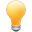Follow us on our fanpages to receive notifications every time there are new articles.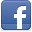Facebook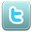Twitter

## 1- What is Transact-SQL?

Transact-SQL is a database procedural programming language. Microsoft's monopoly, used in SQL Server.

Procedural languages are designed to extend SQL's abilities while being able to integrate well with SQL. Several features such as local variables and string/data processing are added. These features make the language Turing-complete.

They are also used to write stored procedures: pieces of code residing on the server to manage complex business rules that are hard or impossible to manage with pure set-based operations.
A Turing Complete system means a system in which a program can be written that will find an answer (although with no guarantees regarding runtime or memory).

## 2- Transact-SQL Overview

T-SQL is organized by each block of statement. A block of statement can embrace another block of statement in it. A block of statement starts by BEGIN and finishes by END. There are many statements in the block, and statements is separated from each other by a semicolon (;).
The structure of the block:
``````
BEGIN
-- Declare variables
-- T-SQL Statements
END;
``````

## 3- Starting with SQL Server Management Studio

In this document, I will instruct you to programme SQLServer on SQL Server Management Studio visual tool.
This is the image of SQL Server Management Studio when you have just opened it. There are some available examples of database when you fully install SQL Server.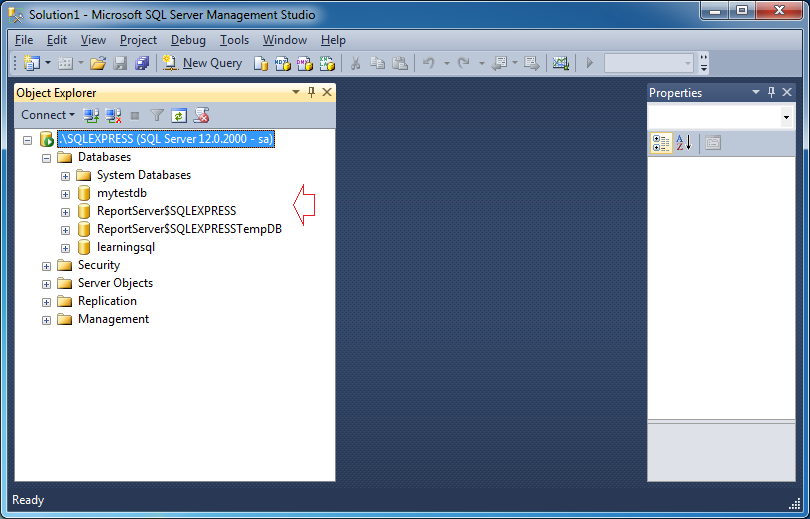You also can create learningsql database, a simple database to be used in some SQL Server documentation on o7planning.org
Right-click on a database, select "New Query" in order to open a query window for this database.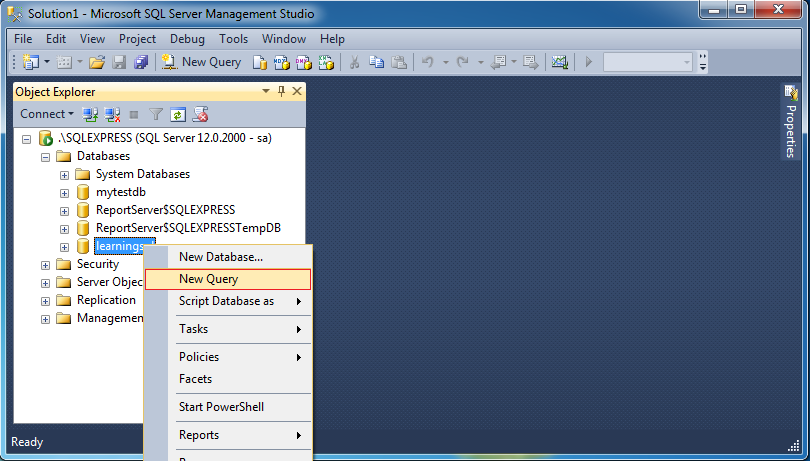You are ready to programme database with SQL Server.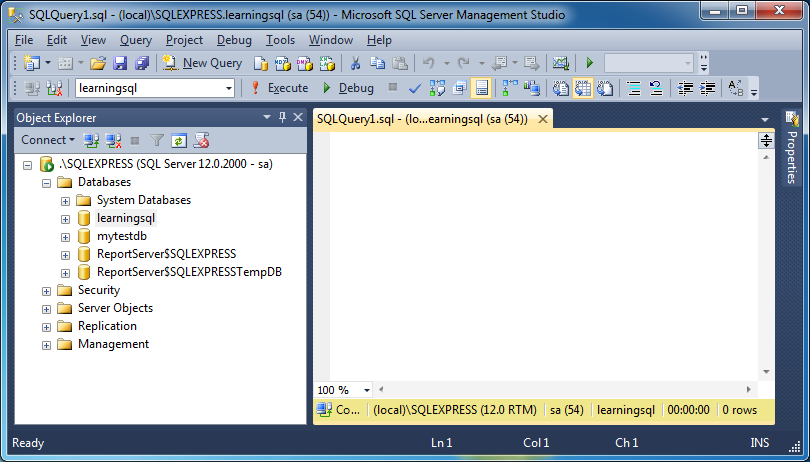Following is a block of simple statements, sum two numbers:
``````
Begin

-- Declaring a variable
Declare @v_Result Int;
-- Declaring a variable with a value of 50
Declare @v_a Int = 50;
-- Declaring a variable with a value of 100
Declare @v_b Int = 100;

-- Print out Console (For developer).
-- Using Cast to convert Int to String
-- Using + operator to concatenate 2 string
Print 'v_a= ' + Cast(@v_a as varchar(15));

-- Print out Console
Print 'v_b= ' + Cast(@v_b as varchar(15));

-- Sum
Set @v_Result = @v_a + @v_b;

-- Print out Console
Print 'v_Result= ' + Cast(@v_Result as varchar(15));

End;
``````
Click on the icon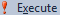in order to execute the block of statement, and see the result on SQL Server Management Studio: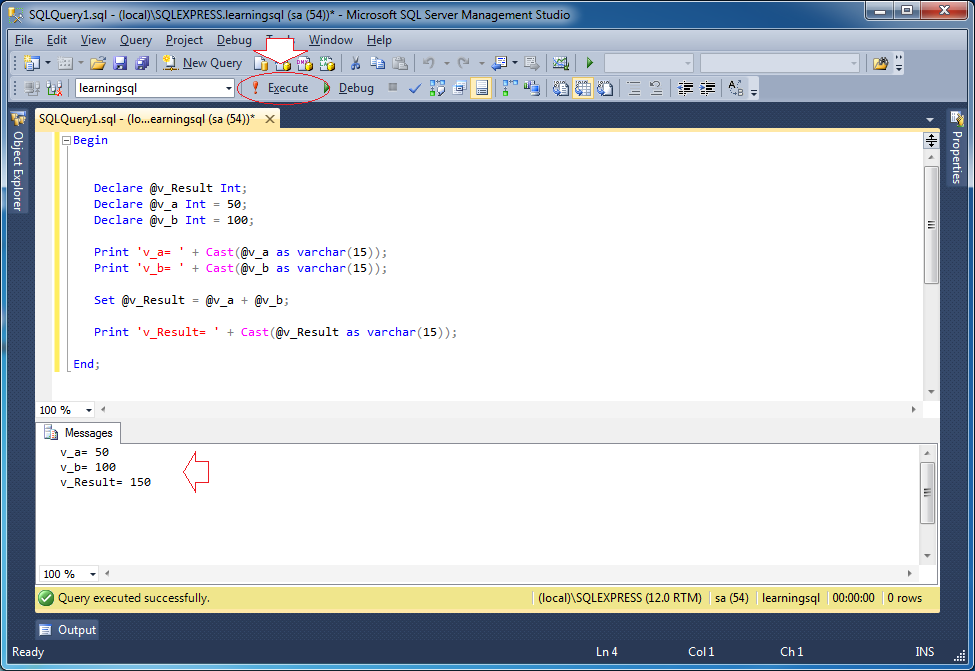## 4- The basic Transact-SQL statements

Here I introduce the overview of basic statements of PL/SQL. You will understand it through examples in the next sections.

### 4.1- Branding statement: If-elsif-else

Syntax:
``````
IF <condition 1> THEN
Job 1;
[ELSIF <condition 2> THEN
Job 2;
]
[ELSE
Job n + 1;
]
END IF;
``````
Example:
Else_If_Example
``````
BEGIN

-- Declare a variable
DECLARE @v_Option integer;
DECLARE @v_Action varchar(30);

SET @v_Option = 2;

IF @v_Option = 1
SET @v_Action = 'Run';
ELSE IF @v_Option = 2
BEGIN
PRINT 'In block else if @v_Option = 2';
SET @v_Action = 'Backup';
END;
ELSE IF @v_Option = 3
SET @v_Action = 'Stop';
ELSE
SET @v_Action = 'Invalid';

-- Logging
PRINT '@v_Action= ' + @v_Action;

END;
``````
The results run the example: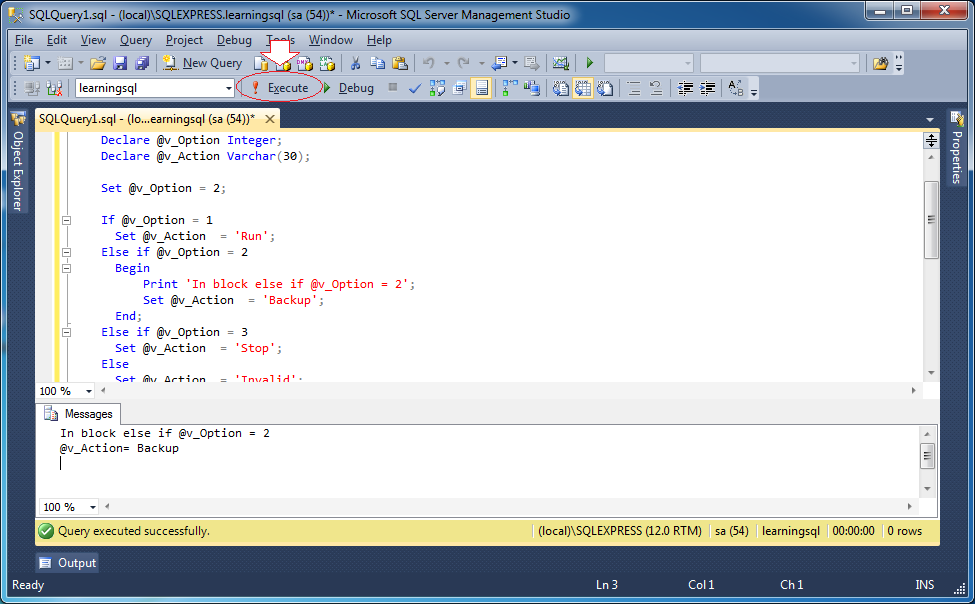### 4.2- WHILE Loop

Syntax:
``````
LOOP
-- Do something here
EXIT WHEN <Condition>;
END LOOP;
``````
In WHILE loop, you can use BREAK in order to exit the loop.
Use CONTINUE statement to skip statements in WHILE block and below it, in order to continue a new loop.
While_Example1
``````
BEGIN

-- Declaring 2 variables x and y.
DECLARE @x integer = 0;
DECLARE @y integer = 10;

-- Step
DECLARE @step integer = 0;

-- While @x < @y
WHILE (@x < @y)
BEGIN

SET @step = @step + 1;

-- Every time loop execute, x increases by 1.
SET @x = @x + 1;
-- Every time loop execute, x decreases by 2.
SET @y = @y - 2;

PRINT 'Step =' + CAST(@step AS varchar(10));
PRINT '@x =' + CAST(@x AS varchar(10)) + ' / @y = ' + CAST(@y AS varchar(10));

END;

-- Write log
PRINT 'x,y = ' + CAST(@x AS varchar(10)) + ', ' + CAST(@y AS varchar(10));

END;
``````
The results run the example: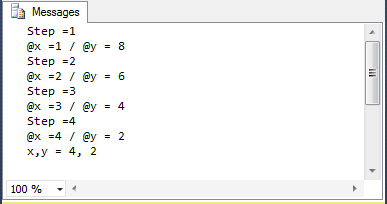BREAK is the statement allowing to exit the loop, following is an example:
While_Example2
``````
BEGIN

-- Declaring 2 variables x and y
DECLARE @x integer = 0;
DECLARE @y integer = 10;

-- Step
DECLARE @step integer = 0;

-- While @x < @y
WHILE (@x < @y)
BEGIN

SET @step = @step + 1;

-- Every time the loop execute, x increases by 1
SET @x = @x + 1;
-- Every time the loop execute, y decreases by 1
SET @y = @y - 2;

PRINT 'Step =' + CAST(@step AS varchar(10));
PRINT '@x =' + CAST(@x AS varchar(10)) + ' / @y = ' + CAST(@y AS varchar(10));

-- If @x > 2 then exit the loop
-- (Although conditions in the WHILE is still true).
IF @x > 2
BREAK;

END;

-- Write log
PRINT 'x,y = ' + CAST(@x AS varchar(10)) + ', ' + CAST(@y AS varchar(10));

END;
``````
The results run the example: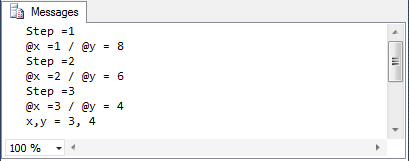CONTINUE statement allows skipping statements below it (in the loop) in order to continue a new loop.
While_Example3
``````
BEGIN

-- Declaring 2 variables x and y.
DECLARE @x integer = 0;
DECLARE @y integer = 10;

-- Step
DECLARE @step integer = 0;

-- While @x < @y
WHILE (@x < @y)
BEGIN

SET @step = @step + 1;

-- Every time the loop execute, x increases by 1
SET @x = @x + 1;
-- Every time the loop execute, x decreases by 2
SET @y = @y - 2;

-- If @x < 3 , then skip the statements below
-- And continue new step
IF @x < 3
CONTINUE;

-- If @x < 3 the statements below 'CONTINUE' will not be run.
PRINT 'Step =' + CAST(@step AS varchar(10));
PRINT '@x =' + CAST(@x AS varchar(10)) + ' / @y = ' + CAST(@y AS varchar(10));

END;

-- Write log
PRINT 'x,y = ' + CAST(@x AS varchar(10)) + ', ' + CAST(@y AS varchar(10));

END;
``````

## 5- Assign values to the variables from the Select statement

Variables can be assigned value from a query. Following is an illustrative example:
Assign_Value_Example
``````
BEGIN

-- Declaring a variable @v_Emp_ID
DECLARE @v_Emp_ID integer = 1;

DECLARE @v_First_Name varchar(30);
DECLARE @v_Last_Name varchar(30);
DECLARE @v_Dept_ID integer;

-- Assgin values to variables
SELECT
@v_First_Name = emp.First_Name,
@v_Last_Name = emp.Last_Name,
@v_Dept_Id = emp.Dept_Id
FROM Employee Emp
WHERE Emp.Emp_ID = @v_Emp_Id;

-- Print out values
PRINT '@v_First_Name = ' + @v_First_Name;
PRINT '@v_Last_Name = ' + @v_Last_Name;
PRINT '@v_Dept_Id = ' + CAST(@v_Dept_ID AS varchar(15));

END;
``````
The results run the example: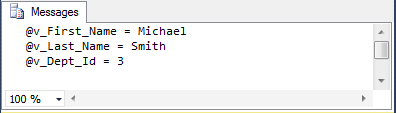## 6- Special data types in T-SQL

### 6.1- TABLE data type (Explicit)

T-SQL allows you to declare a variable of data type TABLE.
Syntax:
``````
-- Define a variable of type TABLE.
-- NOTE: The constraints can also participate in declaration (See example).
Declare @v_variable_name  TABLE  (
Column1 DataType1,
Column2 DataType2
);
``````
Example:
``````
-- Declare a variable of type TABLE.
Declare @v_Table TABLE  (
First_Name Varchar(30),
Last_Name Varchar(30),
Dept_ID Integer,
Salary Float
);

-- The constraints can also participate in declaration:
Declare @v_table TABLE  (
Product_ID Integer IDENTITY(1,1) PRIMARY KEY,
Product_Name  DataType2 NOT NULL Default ('Unknown'),
Price Money CHECK (Price < 10.0)
);
``````
Example: Insert data into a variable of type TABLE.
``````
Insert Into
@v_Table (First_Name, Last_Name, Dept_ID, Salary)
Select Emp.First_Name, Emp.Last_Name, Emp.Dept_Id, 1000
From
Employee Emp
Where Emp.Emp_ID < 4;
``````
You can also Update on the variable of type TABLE:
``````
Update
@v_Table
Set Salary = Salary + 100
Where Dept_Id = 10;
``````
Delete on a variable of type TABLE:
``````
Delete From @v_Table Where Dept_ID = 10;
``````
Query on a variable of type TABLE:
``````
Select * from @v_Table
Where Dept_ID = 10
Order by First_Name;
``````
Example:
``````
BEGIN

DECLARE @v_Emp_ID integer = 1;

-- Declare a variable of type TABLE.
DECLARE @v_Table TABLE (
First_Name varchar(30),
Last_Name varchar(30),
Dept_Id integer,
Salary float DEFAULT 1000
);

-- Using INSERT INTO statement to insert data into @v_Table.
INSERT INTO @v_Table (First_name, Last_Name, Dept_ID)
SELECT
emp.First_Name,
emp.Last_Name,
emp.Dept_Id
FROM Employee Emp
WHERE Emp.Emp_ID < 4;

-- Update @v_Table
UPDATE @v_Table
SET Salary = Salary + 100
WHERE First_name = 'Susan';

-- Query @v_Table.
SELECT
*
FROM @v_Table;

END;
``````
The results run the example: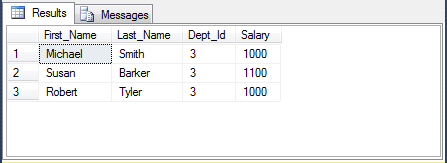### 6.2- TABLE Data type (Implicitly)

T-SQL allows you to declare a variable of type TABLE implicitly. Variable names begin by #.
Table_Example
``````
BEGIN

-- Using SELECT INTO statement to insert data into #v_My_Table.
SELECT
emp.First_Name,
emp.Last_Name,
emp.Dept_Id,
1000 Salary INTO #v_My_Table
FROM Employee Emp
WHERE Emp.Emp_ID < 4;

-- Update #v_My_Table
UPDATE #v_My_Table
SET Salary = Salary + 100
WHERE First_name = 'Susan';

-- Query #v_My_Table.
SELECT
*
FROM #v_My_Table;

END;
``````
The results run the example:## 7- Cursor

### 7.1- What is cursor?

Cursor is a type of structured variable that allows us to process data with various lines. The number of lines is dependent on the data querying statement. During the processing, we manipulate cursor through each data line. This data line is specified by a cursor. By moving the cursor, you can access all rows.

### 7.2- Declaring Cursor

Syntax:
``````
-- ISO Syntax
DECLARE cursor_name [ INSENSITIVE ] [ SCROLL ] CURSOR
FOR select_statement
[ FOR { READ ONLY | UPDATE [ OF column_name [ ,...n ] ] } ]
[;]

-- Transact-SQL Extended Syntax
DECLARE cursor_name CURSOR [ LOCAL | GLOBAL ]
[ FORWARD_ONLY | SCROLL ]
[ STATIC | KEYSET | DYNAMIC | FAST_FORWARD ]
[ READ_ONLY | SCROLL_LOCKS | OPTIMISTIC ]
[ TYPE_WARNING ]
FOR select_statement
[ FOR UPDATE [ OF column_name [ ,...n ] ] ]
[;]
``````

### 7.3- Example with Cursor

Cursor_Example
``````
USE learningsql;

BEGIN

--
-- Declare a variable:
DECLARE @v_Emp_ID integer;
DECLARE @v_First_Name varchar(50);
DECLARE @v_Last_Name varchar(50);

DECLARE @v_Count integer;

-- Declare a CURSOR.
DECLARE My_Cursor CURSOR FOR
SELECT
Emp.EMP_ID,
Emp.FIRST_NAME,
Emp.LAST_NAME
FROM Employee Emp
WHERE Emp.EMP_ID < 3;

-- Open Cursor
OPEN My_Cursor;

-- Move the cursor to the first record.
-- And assign column values to variables.
FETCH NEXT FROM My_Cursor INTO @v_Emp_ID, @v_First_Name, @v_Last_Name;

-- The FETCH statement was successful. ( @@FETCH_STATUS = 0 )
WHILE @@FETCH_STATUS = 0
BEGIN
PRINT 'First Name = '+ @v_First_Name+' / Last Name = '+ @v_Last_Name;

-- Move to the next record.
-- And assign column values to the variables
FETCH NEXT FROM My_Cursor INTO @v_Emp_ID, @v_First_Name, @v_Last_Name;
END

-- Close Cursor.
CLOSE My_Cursor;
DEALLOCATE My_Cursor;

END;
``````
The results run the example: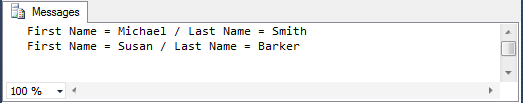### 7.4- Cursor Example (Declare cursor as variable)

Cursor_Example2
``````
USE learningsql;

BEGIN

--
-- Declare a variable:
DECLARE @v_Emp_ID integer;
DECLARE @v_First_Name varchar(50);
DECLARE @v_Last_Name varchar(50);

-- Declaring a cursor variable.
DECLARE @My_Cursor CURSOR;

-- Set Select statement for CURSOR variable.
Set @My_Cursor = CURSOR FOR
SELECT
Emp.EMP_ID,
Emp.FIRST_NAME,
Emp.LAST_NAME
FROM Employee Emp
WHERE Emp.EMP_ID < 3;

-- Open Cursor
OPEN @My_Cursor;

-- Move the cursor to the first line.
-- And assign column values to the variables.
FETCH NEXT FROM @My_Cursor INTO @v_Emp_ID, @v_First_Name, @v_Last_Name;

-- The FETCH statement was successful. ( @@FETCH_STATUS = 0)
WHILE @@FETCH_STATUS = 0
BEGIN
PRINT 'First Name = '+ @v_First_Name+' / Last Name = '+ @v_Last_Name;

-- Move to the next record.
-- And assign column values to the variables.
FETCH NEXT FROM @My_Cursor INTO @v_Emp_ID, @v_First_Name, @v_Last_Name;
END

-- Close Cursor.
CLOSE @My_Cursor;
DEALLOCATE @My_Cursor;

END;
``````
The results run the example:## 8- Exception Handling

When T-SQL programming, errors may occur during runtime. chẳng hạn như lỗi chia cho 0. For example, the error of being divided by 0. Or error when you inserts a record but duplicate value in primary key.
Consider a simple example, divided by 0 error handling.
TryCatch_Example
``````
USE learningsql;

BEGIN

--
-- Declare a variable:
DECLARE @v_a float = 20;
DECLARE @v_b float = 0;
DECLARE @v_c float;
DECLARE @v_Error_Number integer;

-- Use BEGIN TRY .. END TRY to trap errors.
-- If an error occurs in this block
BEGIN TRY

---
PRINT '@v_a = ' + CAST(@v_a AS varchar(15));
PRINT '@v_b = ' + CAST(@v_b AS varchar(15));
-- Divide by 0 error, occurring here.
SET @v_c = @v_a / @v_b;

-- Below this line will not be running.
PRINT '@v_c= ' + CAST(@v_c AS varchar(15));

END TRY
-- BEGIN CATCH .. END CATCH must be placed immediately behind BEGIN TRY .. END TRY.
BEGIN CATCH
-- Error Number.
SET @v_Error_Number = ERROR_NUMBER();
-- Print out error number:
PRINT 'Error Number: ' + CAST(@v_Error_Number AS varchar(15));
-- Error message:
PRINT 'Error Message: ' + ERROR_MESSAGE();
--  The severity of the error:
PRINT 'Error Severity: ' + CAST(ERROR_SEVERITY() AS varchar(15));
-- Error State:
PRINT 'Error State: ' + CAST(ERROR_STATE() AS varchar(15));
-- Line Number:
PRINT 'Error Line: ' + CAST(ERROR_LINE() AS varchar(15));
-- Name of procedure (or function, or trigger).
PRINT 'Error Procedure: ' + ERROR_PROCEDURE();
END CATCH;

END;
``````
The results run the example: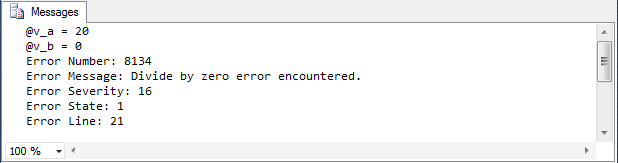Error infomations:
 Function Description ERROR_NUMBER() returns the error number. ERROR_MESSAGE() returns the complete text of the error message. The text includes the values supplied for any substitutable parameters such as lengths, object names, or times. ERROR_SEVERITY() returns the error severity. ERROR_STATE() returns the error state number. ERROR_LINE() returns the line number inside the routine that caused the error. ERROR_PROCEDURE() returns the name of the stored procedure or trigger where the error occurred.

## 9- Function

Like procedure, function is also a group of PL/SQL statements that play some role. Unlike procedure, a function will return a value right on its call.

Functions are also stored on database in form of Store procedure.
Syntax create function.
``````
-- function_name:
-- argument:
-- mode:  INPUT, OUTPUT,  default INPUT
-- datatype:

CREATE FUNCTION <function_name>
(
[
@argument1 datatype1 [mode1] ,
@argument2  datatype2 [mode2],
...
]
)
RETURNS  datatype
AS
BEGIN
-- Declare variables
-- Statements
-- Return value
END;
``````
Example:
``````
-- Function with parameters
CREATE FUNCTION Sum_Ab(a Integer, b Integer)
RETURNS Integer
AS
Begin
return a + b;
End;

-- Function without parameters
CREATE FUNCTION Get_Current_Datetime()
RETURNS Date
AS
Begin
return CURRENT_TIMESTAMP;
End;
``````
Drop Function:
``````
-- Drop Function

DROP FUNCTION <function_name>;

-- For example:

DROP FUNCTION My_Function;
``````
Function Example:
Here is an example of creating your first function with SQL Server:
1.  Create a function (Function)
2.  Compile this function
3.  Running function
``````
-- Check the existence of the function
-- If it did exist, should drop it in order to create a new one.
IF OBJECT_ID(N'dbo.My_Sum', N'FN') IS NOT NULL
DROP FUNCTION My_Sum;
GO

CREATE FUNCTION My_Sum (@p_a float, @p_b float)
RETURNS float
AS
BEGIN

-- Declaring a variable type of Float
DECLARE @v_C float;

-- Assign value for v_C
SET @V_C = @p_A + @p_B;

-- Return value.
RETURN @v_C;

END;
``````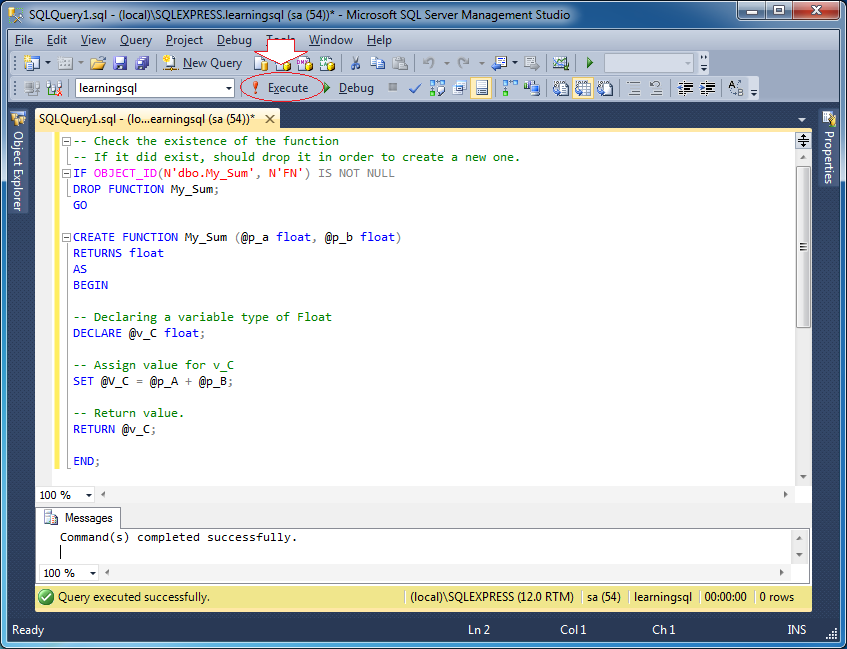Click the iconto compile the function.
The function you have created is a simple function. It returns a Scalar value. You can see that it has been created on SQLServer Management Studio: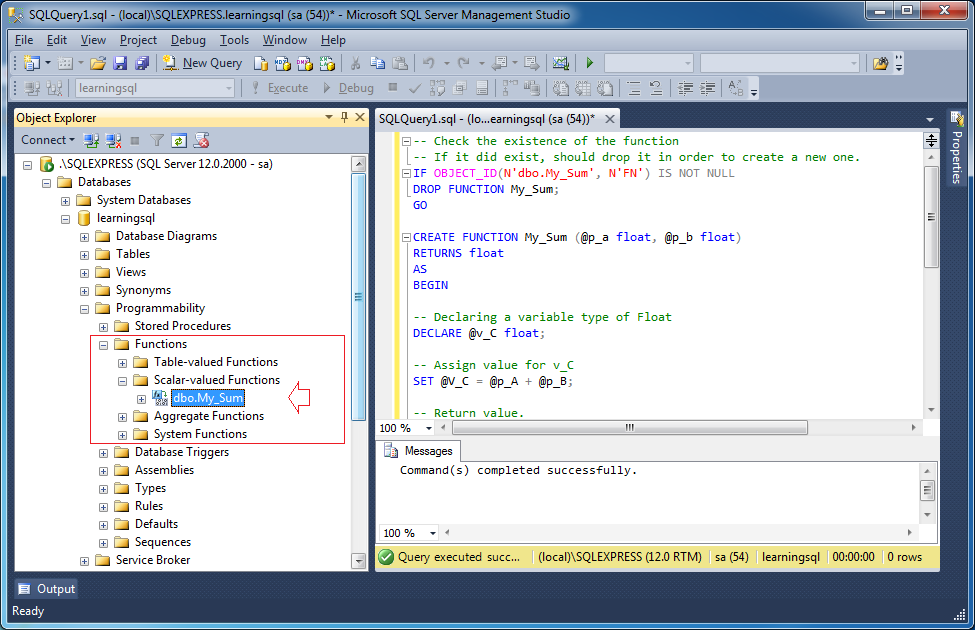You can test function by right-clicking on the function, select:
• Script function as -> SELECT to -> New Query Editor Window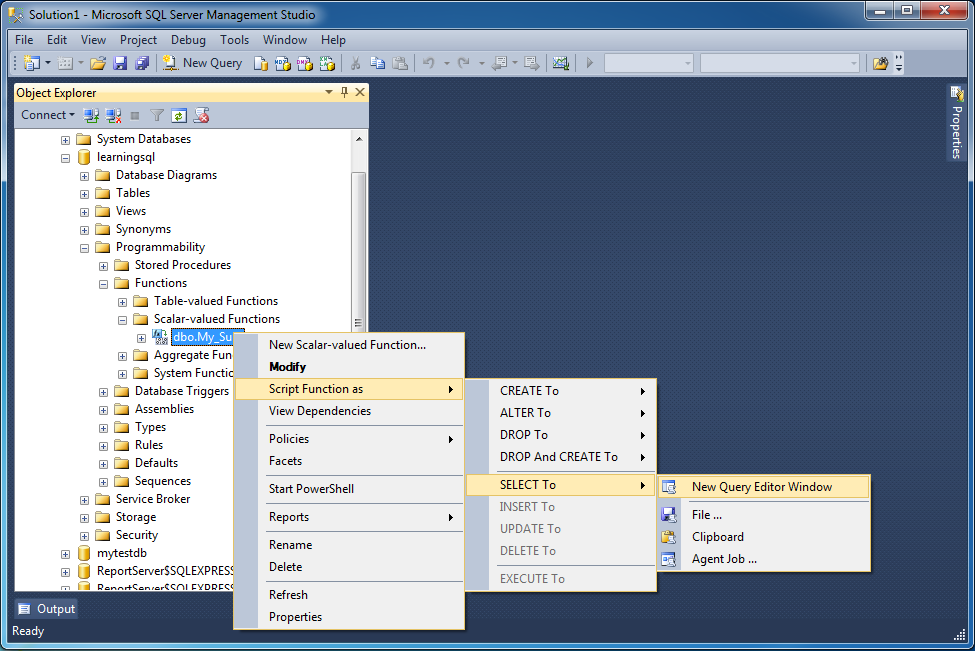A test window is open, you can modify the parameter values: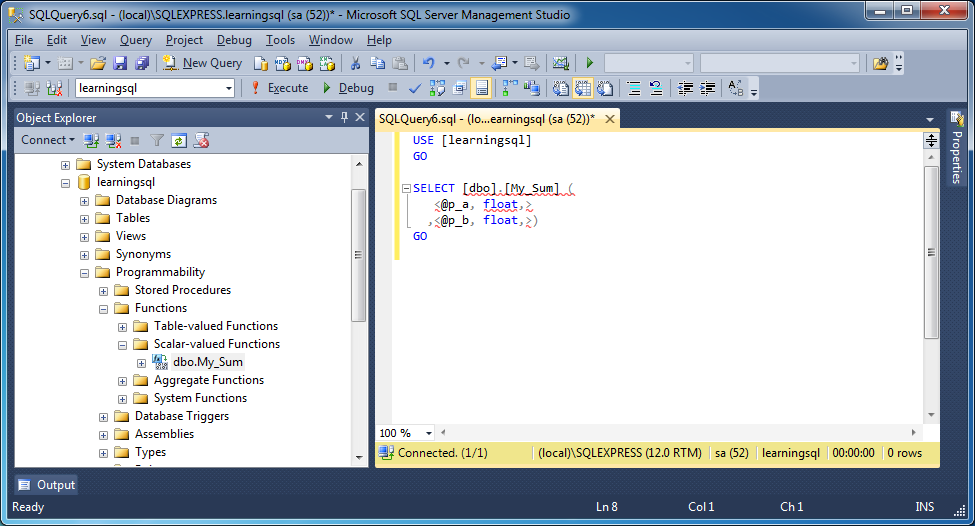Change the parameter values and press the execute button.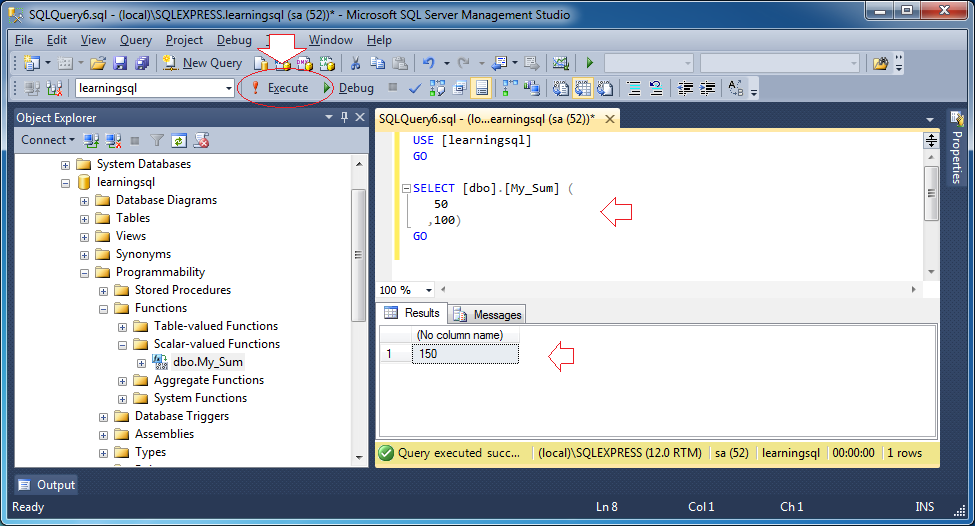The function can participate in SELECT statements
``````
SELECT
acc.account_id,
acc.cust_id,
acc.avail_balance,
acc.pending_balance,
dbo.MY_SUM(acc.avail_balance, acc.pending_balance) balance
FROM account acc;
``````
Query Result: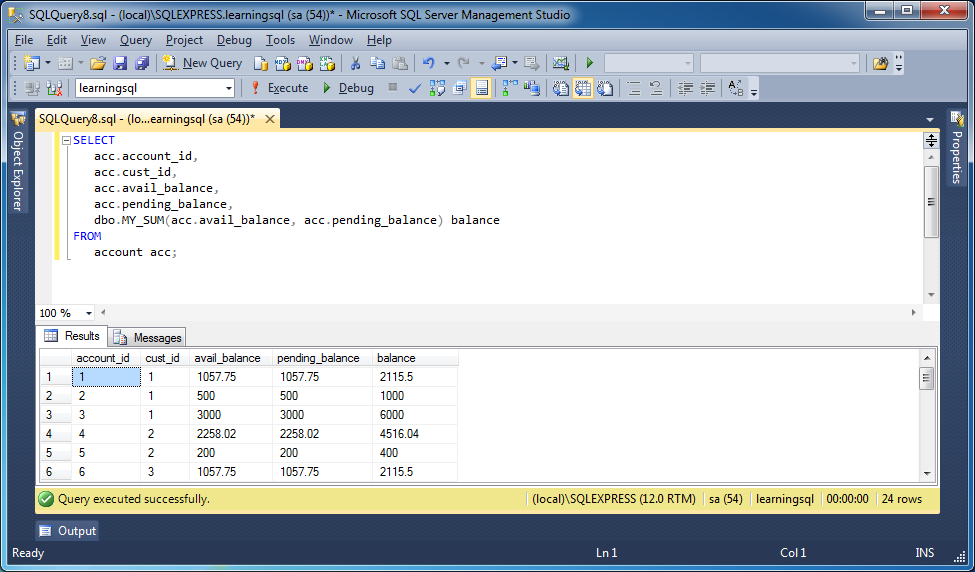## 10- Procedure

A group of statements performing some function can be gathered in a procedure for the purpose of increasing the capability of handling, Reuse, security, data safety, and utility in development.
Procedure can be saved on database as a object of database and ready for reuse. Procedure then is called Store procedure. To Store procedure,after Store procedure is saved, it is translated into p-code so its performing capability is raised.
Procedures do not return values directly as a function. However it may have 0 or more output parameters.
The syntax to create a procedure:
``````
-- procedure_name:
-- argument:
-- mode:  input type: INPUT or OUTPUT, default is INPUT
-- datatype:
-- Note: The procedure parameters can put in an (), or unnecessary.

CREATE PROCEDURE <procedure_name>
[
argument1  datatype1 [mode1]  ,
argument2   datatype2 [mode2]  ,
...
]
AS
BEGIN
-- Declare variables.
-- Statements ..
END;

-- OR:

CREATE PROCEDURE <procedure_name>
(
[
argument1  datatype1 [mode1]  ,
argument2   datatype2 [mode2]  ,
...
]
)
AS
BEGIN
-- Declare variables.
-- Statements ..
END;
``````
Example:
``````
-- Procedure without parameters.
CREATE Procedure Do_Something
AS
Begin
-- Declare variables here.
Declare @v_a Integer;
-- Do something here
-- ....
End;

-- Procedure with parameters
--  1 input parameter and 2 output parameters

CREATE Procedure Do_Something (@p_Param1 Varchar(20),
@v_Param2 Varchar(50) OUTPUT )
AS
Begin
-- Declare variables
Declare @v_a Integer;

-- Do something here.
-- ...
End;
``````
Drop procedure:
``````
-- Drop Procedure:

DROP PROCEDURE <Procedure_Name>
``````
The steps for a procedure: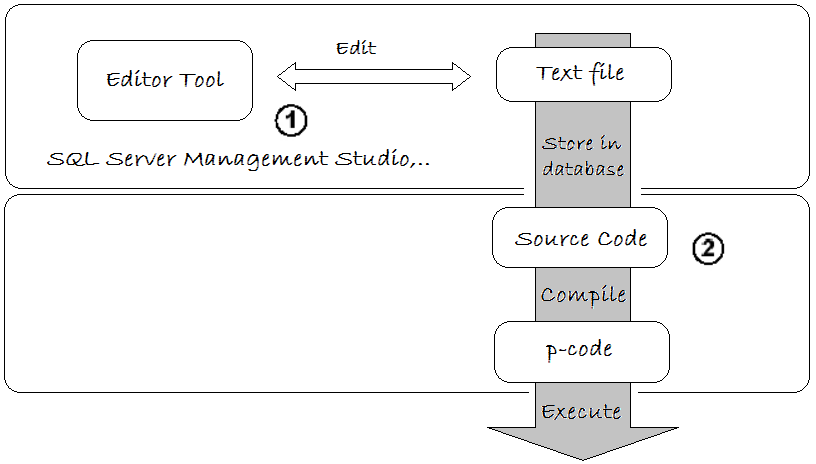Procedure Example:
Here I create a simple procedure with a input parameter named @p_Emp_ID and three output parameters including @v_First_Name, @v_Last_Name, @v_Dep_ID.
Get_Employee_Infos
``````
-- Drop procedure Get_Employee_Infos if it already exists.
-- (To enable recreate)
IF OBJECT_ID(N'dbo.Get_Employee_Infos', N'P') IS NOT NULL
DROP PROCEDURE Get_Employee_Infos;
GO

-- Procedure with input parameter: p_Emp_Id
-- And output: v_First_Name, v_Last_Name, v_Dept_Id.
CREATE PROCEDURE Get_Employee_Infos (@p_Emp_Id integer
, @v_First_Name varchar(50) OUTPUT
, @v_Last_Name varchar(50) OUTPUT
, @v_Dept_Id integer OUTPUT)
AS
BEGIN
-- Use the Print command to print out a string (for programmers).
-- Use Cast to convert Integer to string (Varchar).
-- Use the + operator to concatenate two strings.
PRINT 'Parameter @p_Emp_Id = ' + CAST(@p_Emp_ID AS varchar(15));
--
-- Query data from the table and assign values to variables.
--
SELECT
@v_First_Name = Emp.First_Name,
@v_Last_Name = Emp.Last_Name,
@v_Dept_Id = Emp.Dept_Id
FROM Employee Emp
WHERE Emp.Emp_Id = @p_Emp_Id;

--
-- Log (For developers).
--
PRINT 'Found Record!';
PRINT ' @v_First_Name= ' + @v_First_Name;
PRINT ' @v_Last_Name= ' + @v_Last_Name;
PRINT ' @v_Dept_Id= ' + CAST(@v_Dept_Id AS varchar(15));

END;
``````
Click the iconto compile the procedure.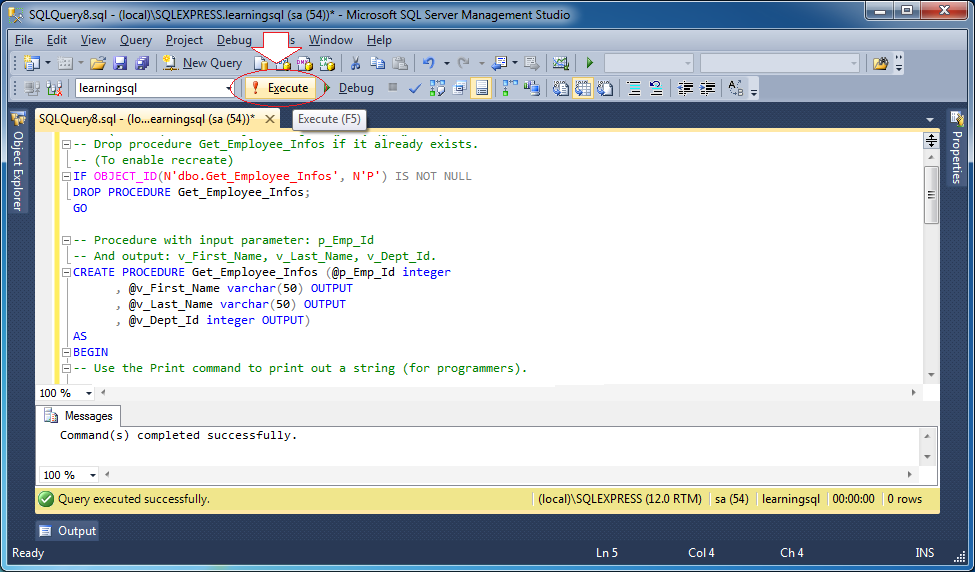After procedure is created, you can see it on SQL Server Management Studio: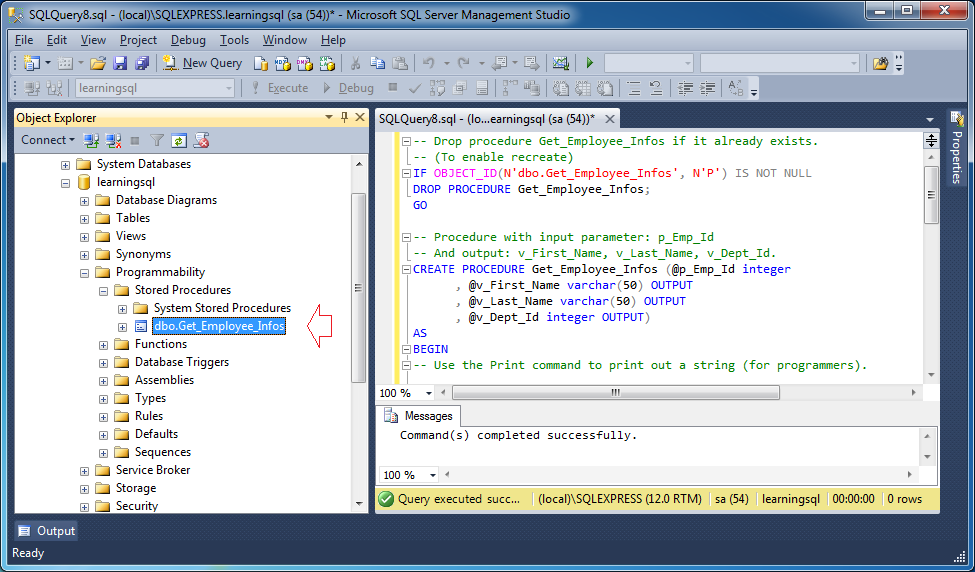Test procedure
In programme, testing procedure and detect error are very important. Right-click on the procedure you want to test, select:
• Script stored Procedure as -> EXECUTE to -> New Query Editor Window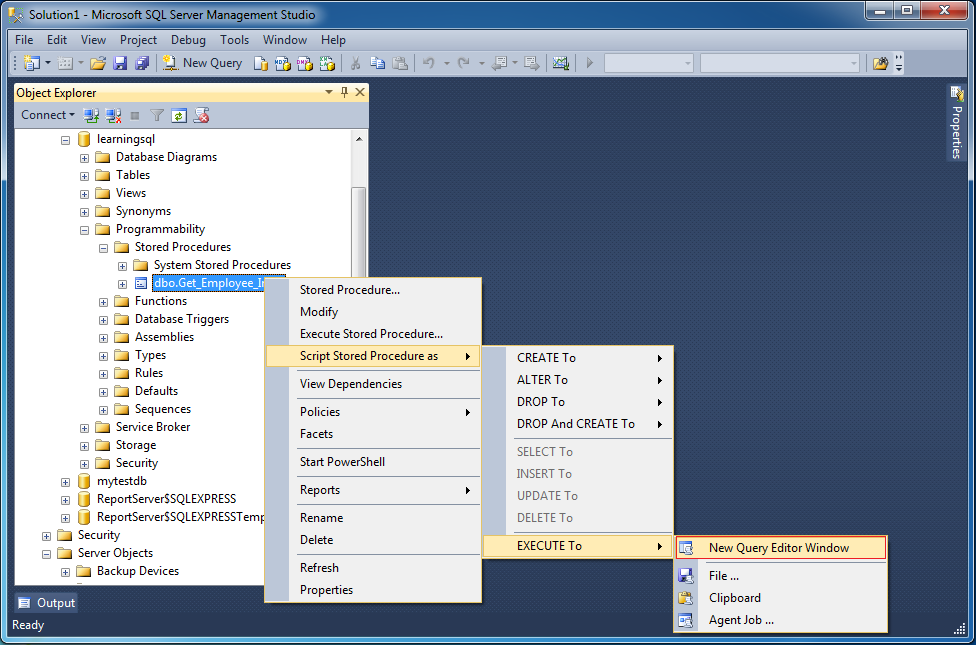Test script are created (Default) as shown below: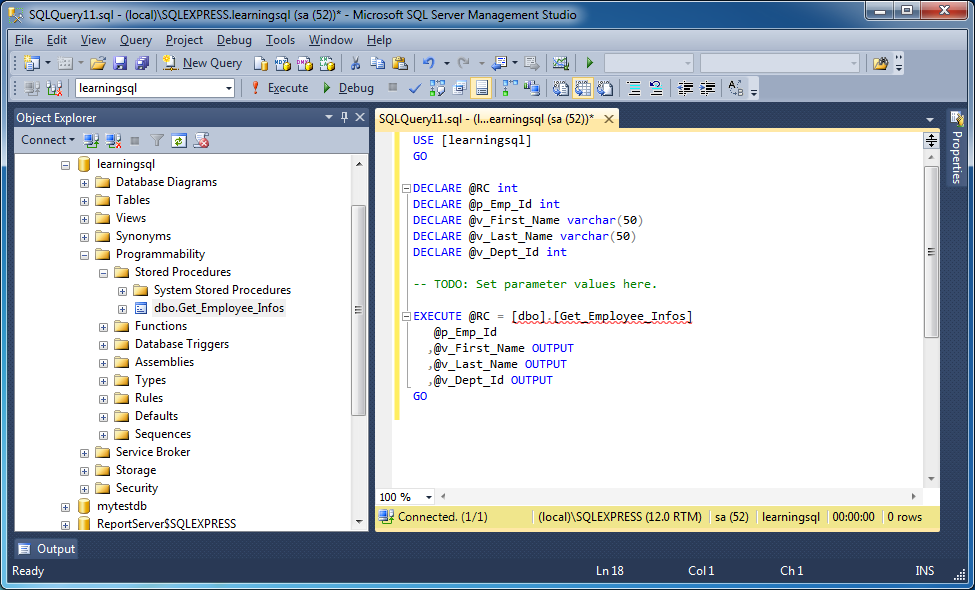Set values for the input parameters: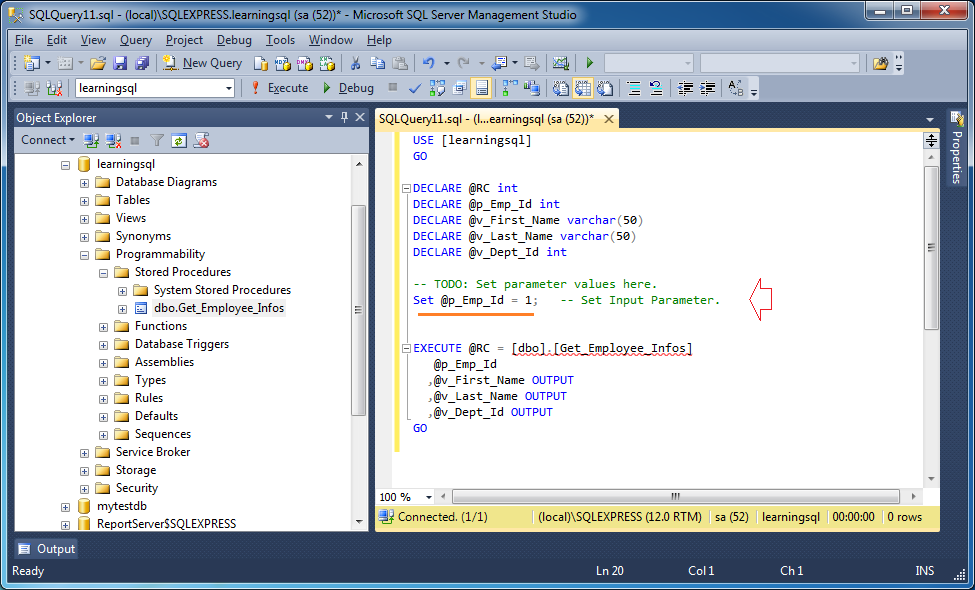Press the button to execute the procedure: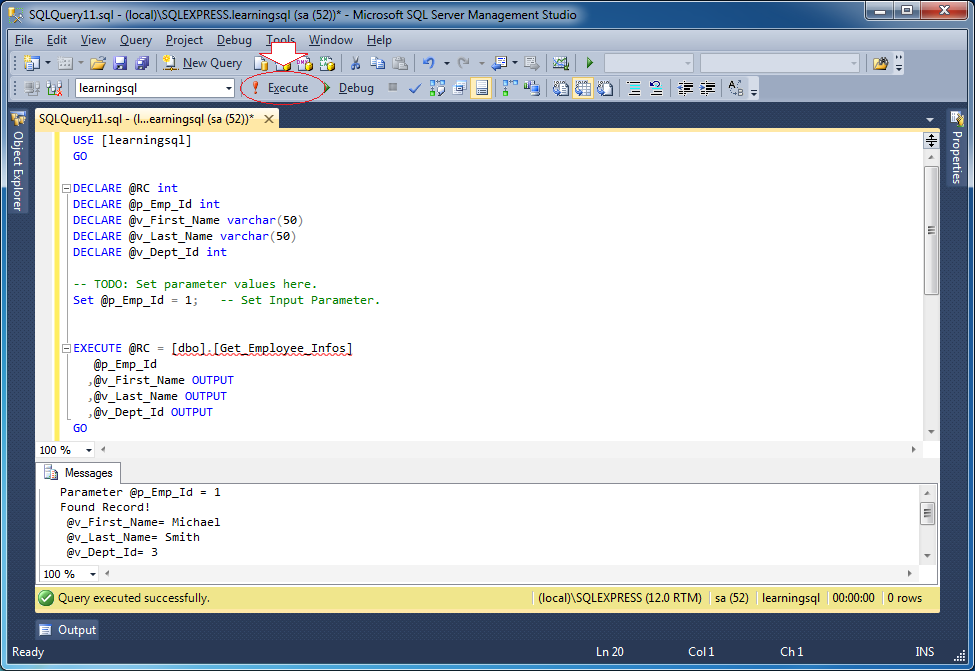## 11- Transaction

### 11.1- Why should the transaction processing?

Transaction is a important concept in SQL. Let's see a situation:

In a banking transaction, A person transfers a sum of 100 dollar to B person. At that time, in Database there are two manipulations:
1. Debit 100 dollar from the account of A person
2. Credit 100 dollar from the account of B person
What would happen if only one manipulation is successful?
See another example:
When you add a student to a class, you need to update the number of students. If the insertion of students' information fails, but the number of students is added 1, the completeness of data is broken.
``````
-- Insert into Student table.
Insert into Student (Studen_Id, Student_Name, Class_ID)
values (100, 'Tom', 1);

-- Update Studen_Count.
Update Class_Table
set Student_Count = Student_Count + 1
Where Class_Id = 1;
``````
Transaction is considered successful if all statement units is executed successfully. On the contrary, one of statement units have error, the whole transaction has to rollback to the initial status.

### 11.2- Declaring and using transactions

Related statements:
1. Begin transaction:
• begin tran / begin transaction
2. Finish transaction:
• commitcommit tran / commit transaction
3. Rollback transaction:
• rollback / rollback tran / rollback transaction
4. Mark a savepoint in transaction: save transaction name_of_savepoint
5. @@trancount variable: shows the number of transactions is being executed (has not been finished with rollback or commit) in the current connection.
Notes:
1. Rollback tran + name_of_savepoint statement helps rollback the transaction to the corresponding position of savepoint (without the effect of finishing the transaction), locks set up will be unlocked when manipulations in rollbacked part are executed.
2. When declaring an explicit transaction, you must ensure that it is rollbacked then or committed to explicit, if not, the transaction will go on existing and occupy resources, preventing the execution of other transactions.
3. Rollback statement only helps rollback transactions on database (insert, delete, update). Other statements, for example assign statement, are not influenced by rollback statement.
Example:
Transaction_Example1
``````
BEGIN

-- In this example the accounts ACCOUNT_ID = 1, 2 actually exists in DB
-- In fact you can write statements to check before the start of transaction
--
-- account A (Already guarantees exist in DB)
DECLARE @Account_Id_A integer = 1;
-- account B (Already guarantees exist in DB)
DECLARE @Account_Id_B integer = 2;
-- Amount
DECLARE @Amount float = 10;
-- Bank
DECLARE @Execute_Branch_Id integer = 1;

-- Write out transaction Count.
-- In fact, at this time there is no transaction yet
PRINT '@@TranCount = ' + CAST(@@Trancount AS varchar(5));

PRINT 'Begin transaction';

-- Begin transaction
BEGIN TRAN;

-- Error trapping.
BEGIN TRY
--
-- Subtract \$10 from account A
UPDATE Account
SET AVAIL_BALANCE = AVAIL_BALANCE - @Amount
WHERE Account_Id = @Account_Id_A;
--
-- Insert transaction info  into Acc_Transaction table.
INSERT INTO ACC_TRANSACTION (TXN_DATE, FUNDS_AVAIL_DATE, TXN_TYPE_CD,
ACCOUNT_ID, AMOUNT, EXECUTION_BRANCH_ID)
VALUES (CURRENT_TIMESTAMP, CURRENT_TIMESTAMP, 'CDT',
@Account_Id_A, -@Amount, @Execute_Branch_Id);
--
-- Add \$10 to Account B.
UPDATE Account
SET AVAIL_BALANCE = AVAIL_BALANCE + @Amount
WHERE Account_Id = @Account_Id_B;
--
-- Insert transaction info  into Acc_Transaction table.
INSERT INTO ACC_TRANSACTION (TXN_DATE, FUNDS_AVAIL_DATE, TXN_TYPE_CD,
ACCOUNT_ID, AMOUNT, EXECUTION_BRANCH_ID)
VALUES (CURRENT_TIMESTAMP, CURRENT_TIMESTAMP, 'CDT', @Account_Id_B,
@Amount, @Execute_Branch_Id);
-- Commit transaction
IF @@Trancount > 0
PRINT 'Commit Transaction';
COMMIT TRAN;

END TRY
-- If there are errors Catch block will be execute.
BEGIN CATCH
PRINT 'Error: ' + ERROR_MESSAGE();
PRINT 'Error --> Rollback Transaction';
IF @@Trancount > 0
ROLLBACK TRAN;
END CATCH;

END;
``````
The results run the example: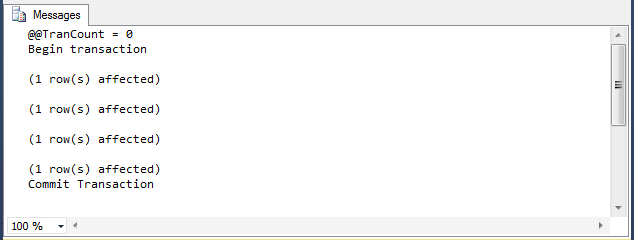## 12- Trigger

Document of Trigger is separate, you can see the instruction at:

#### View more Tutorials:

Maybe you are interested

These are online courses outside the o7planning website that we introduced, which may include free or discounted courses.